Thank you for visiting nature.com. You are using a browser version with limited support for CSS. To obtain the best experience, we recommend you use a more up to date browser (or turn off compatibility mode in Internet Explorer). In the meantime, to ensure continued support, we are displaying the site without styles and JavaScript.

# Observing fermionic statistics with photons in arbitrary processes

## Abstract

Quantum mechanics defines two classes of particles-bosons and fermions-whose exchange statistics fundamentally dictate quantum dynamics. Here we develop a scheme that uses entanglement to directly observe the correlated detection statistics of any number of fermions in any physical process. This approach relies on sending each of the entangled particles through identical copies of the process and by controlling a single phase parameter in the entangled state, the correlated detection statistics can be continuously tuned between bosonic and fermionic statistics. We implement this scheme via two entangled photons shared across the polarisation modes of a single photonic chip to directly mimic the fermion, boson and intermediate behaviour of two-particles undergoing a continuous time quantum walk. The ability to simulate fermions with photons is likely to have applications for verifying boson scattering and for observing particle correlations in analogue simulation using any physical platform that can prepare the entangled state prescribed here.

## Introduction

A particularly striking departure from classical physics in quantum mechanics is the existence of two fundamental particle classes characterised by their behaviour under pairwise exchange (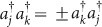). For example an arbitrary number of bosons (obeying Bose-Einstein statistics: ‘+’) can occupy a single quantum state, leading to bunching1 and Bose-Einstein condensation; whereas fermions (obeying Fermi-Dirac statistics: ‘−’) are strictly forbidden to occupy the same state via the Pauli-exclusion principle2. Behaviour intermediate between bosons and fermions can also be considered; particles confined to two dimensions known as anyons3 are predicted to exhibit intermediate behaviour with fractional exchange statistics (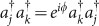, 0 < ϕ < π)—a concept applied in superconductivity4 and used to explain the fractional quantum Hall effect5.

Here we show that by tuning one phase parameter, entanglement and a single experimental setup can exactly simulate the measurement statistics of an arbitrary mode transformation A (Fig. 1 (a)) of N identical fermions, bosons or particles with fractional statistics. It is well known that the symmetric and anti-symmetric states |ψ±〉 = |H1 |V2 ± |V1 |H2 of two polarised photons input into a 50% reflectivity beam splitter exhibits fermion-like anti-bunching and boson-like bunching, respectively6. Furthermore, intermediate behaviour for a beamsplitter has been observed by controlling a phase to tune between the two Bell states |ψ+〉 and |ψ7,8. It is less widely appreciated that the resulting detection statistics can also be exactly mimicked with two photon path-number entangled NOON states9; however this behaviour does not generalise to processes other than beamsplitters, the simplest example being the identity operation for which bunching always occurs regardless of the phase of the NOON state. The approach we present shares N-partite, N-level entanglement, parameterised by phase ϕ, across N copies of A (Fig. 1 (b)).

After presenting the scheme in the case of two particles in an arbitrary linear evolution, we experimentally demonstrate the simulation of arbitrary particle statistics with a complex photonic network—a continuous time quantum walk10,11. We achieve the required entangled state with post-selected polarisation entanglement shared across the TE and TM modes of an evanescently coupled waveguide array. This enables us to directly observe the evolution of symmetric and anti-symmetric two particle wave functions in a coupled oscillator Hamiltonian by switching the phase of the entangled state between 0 and π. Mixed-symmetric quantum walk behaviour is also observed by tuning the entangled state's phase between 0 and π, corresponding to behaviour intermediate between bosons and fermions. We note the fermion simulation directly maps to the dynamics of two excitations in a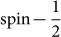chain consisting of ten sites: Using the Jordan-Wigner transformation, excitations correspond to fermion creation operators and the vacuum to the ground state of each spin12. We finally provide a mathematical demonstration of our scheme in the case of an arbitrary number of particles evolving through any linear Hamiltonian. It is important to note that neither the two-particle case, nor the N-particle generalization, require any post-selection and are, in princlple, scalable.

## Results

Exchange statistics refer to a wave-function acquiring phase ϕ after interchanging two indistinguishable quantum particles. Occupation of labeled modes j are modelled by annihilation operators aj and creation operators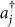that obey the commutation relations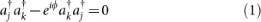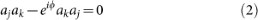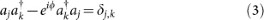for j < k. In three dimensions particles are either bosons (ϕ = 0) or fermions (ϕ = π) and successive swapping is represented by the permutation group while the correlated detection statistics are associated respectively to the permanent and determinant of a given matrix13. In one- and two-dimensions anyons3 can exist where swapping two identical anyons ω times (represented by the braid group) results in the combined wave-function acquiring phase ϕ = ωθ where 0 < θ < π and the positive (or negative) winding number ω keeps track of the number of times two anyons braid in trajectory space with clockwise (or anti-clockwise) orientation4.

The quantum correlation function for two identical non-interacting particles (input into j and k of A and detected at outputs r and q) is given by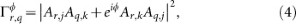for bosons14 (ϕ = 0) and fermions13 (ϕ = π). Here, the process A is represented by a matrix whose element Ar,j is the complex transition amplitude from j to r. Non-classical behaviour is evident in the constructive and destructive interference between typically complex terms inside the |·|2, dictated by ϕ = ωθ. By defining fractional exchange algebra (1–3) for 0 < θ < π and some integer winding number ω, we can observe the effect of fractional exchange statistics on quantum interference between two indistinguishable anyons undergoing braiding operations dependent upon A; Eq. (4) represents the resulting quantum interference between two abelian anyons (0 < θ < π) in superposition of braiding ω times and not braiding at all. To see this we define without loss of generality the input labels by j < k and the output labels by r < q and consider two identical anyons created at modes j and k, transformed according to A and detected at modes r and q. Correlated detection at r and q reveals two indistinguishable possibilities that correspond to the two terms in Eq. (4): A maps the anyon at j to mode r and the anyon at k to mode q with amplitude Ar,j Aq,k; or A maps the anyon at j to mode q and the anyon at k to mode r via ω clockwise braids with fractional phase ϕ and amplitude Aq,jAr,k. From this definition, we use relations (1–3) to derive expression (4) for 0 < θ < π and ϕ = ωθ (see Supplementary Information).

To physically simulate quantum interference given by Eq. (4), we use two copies of A, denoted Aa and Ab, together with bi-partite entanglement of the form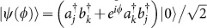where creation operators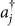and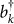are associated to identical networks Aa and Ab respectively; creation operator sub-indices denote the modes on which the creation operators act. Once |ψ(ϕ)〉 is generated, the class of input entangled particles becomes irrelevant; only one particle is present in each of Aa and Ab, therefore no further quantum interference between particles will occur. This implies application with other quantum platforms, using for example entangled fermions to simulate Bose-Einstein quantum interference. Evolution of the two particle state through the tensor product of transformations is therefore modelled by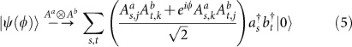It follows that correlated detection probability across outputs r of Aa and q of Ab simulates Eq. (4) according to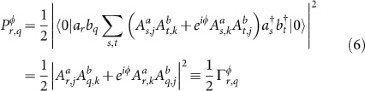where the 1/2 pre-factor is due to normalisation of the initial entanglement. Since the phase of the entangled state can be defined as ϕ = ωθ, this method simulates the interference statistics of two anyons of fractional phase θ that have not braided, with two anyons also characterised by θ that have braided ω times (equivalently anyons that are in a superposition of braiding ω1 and ω2 times for ϕ = (ω1 − ω2)θ—the ideal statistics are the same).

We experimentally simulated Eq. (4) for arbitrary ϕ in a coupled oscillator Hamiltonian equivalent to sharing entanglement across two continuous time quantum walks, realised with arrayed waveguides10 (see Supplementary Information). We measured the correlations of the polarisation entangled state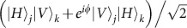shared across two copies of a process A: Aa and Ab, realised as the TE and TM modes of a waveguide array fabricated in silicon oxynitride (SiOxNy) (Fig. 2 (b), see also Methods). The waveguides and butt-coupled polarisation maintaining fibres (PMFs) are birefringent to ensure negligible cross-talk between horizontal and vertical polarisations. Detection statistics of the output photons in the {|H〉, |V〉} basis were analysed using polarising beam splitters (PBS) and pairs of multi-mode fibre coupled avalanche photo-diode single photon counting modules (SPCM) (Fig. 2 (c)). By inputting the state (|Hj + |Vj)(|Hk + e−iϕ|Vk)/2 and detecting one |H〉 photon and one |V〉 photon at any pair of outputs of Aa and Ab we post-selected the input state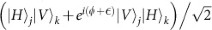, with a measurable phase off-set(from birefringence of PMF and the waveguide circuit). This phasewas compensated using a polarisation phase rotation (Fig. 2 (a)). Note that because of this compensation, the approach described is immune to birefringence of the 2 m PMF and input waveguides.

We measured two sets of correlation matrices for two different inputs: immediate neighbouring waveguides in the centre of the array (j, k) = (−1, 0) (Fig. 3); and next but one neighbouring waveguides (j, k) = (−1, 1), with vacuum input in waveguide 0 (Fig. 4). Each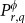entry is the probability to detect a horizontal and vertical polarised photon at outputs r and q, respectively. Five cases are shown for ϕ = 0, π/4, π/2, 3π/4, π (left: (a), (c), (e), (g), (i)). The corresponding ideal correlation matrices given by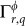in Eq. (4) are also plotted (right: (b), (d), (f), (h), (j)). For the (j, k) = (−1, 0) input (Fig. 3) we observe the expected bunching behaviour of bosons for ϕ = 0 which is contrasted with the observation of the Pauli-exclusion in the diagonal elements of the matrices for ϕ = π. We note that a form of exclusion on the diagonal entries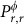would also occur for experiments with hard core bosons, but the remaining correlation matrix would still be dependent upon Bose-Einstein exchange statistics. The spectrum of phase 0 < ϕ < π exhibits a variable Pauli-exclusion and a continuous transition between simulation of Bose-Einstein and Fermi-Dirac quantum interference. In the bosonic case, we observe a less pronounced bunching behaviour for the (j, k) = (−1, 1) input (Fig. 4 (a)) than for the (j, k) = (−1, 0) input (Fig. 3 (a)), as expected14. This behaviour is inverted in the fermionic case, since the particels tend to propagate to the same peak of the walk (while still not occupying the same waveguide) for the (j, k) = (−1, 1) input (Fig. 4 (i)) and tend to propagate in different peaks for the (j, k) = (−1, 0) input (Fig. 3 (i)). This is the result of the different phases in the Hamiltonian that describes the quantum walk evolution.

Agreement between the ideal implementation of our scheme, with perfect realisations of Aa and Ab and the data in Figs. 3,4 is quantified in Table I using the similarity S between the ideal correlation matrix Γϕ and the measured distribution of detection statistics Pϕ: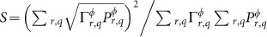, a generalisation of the average (classical) fidelity between two probability distributions that ranges from 0% (anti-correlated distributions) to 100% for complete agreement. We also calculated S when we deliberately made the photons distinguishable by temporally delaying one with respect to the other. This enabled physical simulation of the classical correlations ΓC = |Ar,jAq,k|2 + |Ar,kAq,j|2 that are independent of particle statistics. For these measurements, we found agreement with the ideal model of S = 97.4 ± 0.7% and S = 97.5 ± 0.6% for inputs (−1, 0) and (−1, 1) respectively. These data indicate that Aa and Ab are near identical while keeping crosstalk between horizontal and vertical polarisation to a minimum. While non-unit similarity (S = 100%) in Table I is attributed to non-perfect entanglement generation, the high level of agreement between the ideal and measured cases indicates accurate quantum simulation of arbitrary quantum interference in a uniform coupled oscillator Hamiltonian.

Using multi-partite entanglement allows simulation of quantum interference of an arbitrary number N of identical non-interacting particles. N indistinguishable particles with exchange statistics ϕ, subjected to mode transformation A at inputs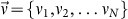, are correlated due to quantum interference across outputs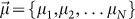according to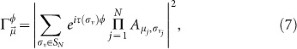where σν is an element of the permutation group SN acting onand τ (σν) is the minimum number of neighbouring swaps that maps σν to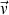;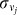denotes the element j of σν. For ϕ = 0 and ϕ = π, Eq. (7) is the modulus-square of the permanent and determinant respectively of an N × N subset of A. For 0 < ϕ < π Eq. (7)—which is the modulus-square of the imminent of the N × N subset of A—is derived in the Supplementary Information. The multi-particle correlation function Eq. (7) is simulated by subjecting the generalised N-partite, N-level entangled state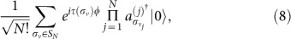to N copies of A such that one particle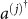is launched into each copy A(j). For example, for inputs j, k, l and N = 3, the required entangled input state is equivalent to three entangled qutrits, shared across three copies of A denoted Aa, Ab and Ac: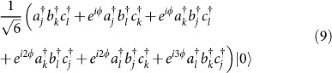N-fold coincidence detection across outputs μj of A(j) equals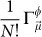. Note as for the case with two particles, the 1/N! pre-factor can be canceled by considering all N! permutations of the outputs, therefore directly simulating quantum interference of N bosons (ϕ = 0) or N fermions (ϕ = π). For example, in the 2-particle case, the 1/2 pre-factor in Eq. (6) is canceled by summing both the detection outputs of a particle at r on Aa, q on Ab and at r on Ab, q on Aa. All other values of ϕ allow continuous transition between Fermi and Bose quantum interference. Note that the approach of initialising the simulation with a pre-symmetrized (or pre-antisymmetrized) state is in the spirit of first quantised quantum simulation15, for which quantum algorithms have been designed to efficiently generate the required states16,17 (see Supplementary Information). In a photonic realization of the proposed sheme, the required N-particle entangled state can be generated using additional photons and feedforward to implement quantum gates following the KLM scheme18.

## Discussion

Correlations resulting from quantum interference of multiple indistinguishable non-interacting bosons in large linear optical circuits cannot be efficiently simulated with classical computers19,20,21. Large scale repeated quantum interference is achievable with photons in waveguides11,22,23,24, which is likely to provide the platform for the realisation of such a boson scattering device, to realise an engineered system that can outperform the limits of classical computation. A major issue will be verifying boson scattering correlations that exceed the capabilities of conventional computers. In contrast, the analogous situation for non-interacting fermions is efficient with classical computers (currently it is not as straightforward to perform quantum interference of fermions2,25 as it is with photons in integrated optics). The ability to tune continuously between tractable and intractable phenomena with a single setup could be essential for verification of bosonic scattering.

Other possible applications for simulating particle statistics with photonics include preparing appropriately symmetrized states in quantum chemistry simulations15 and combinatorics, where exchange statistics have been shown to alter mathematical graph structure26—three-dimensional waveguide architectures27 may be important here. Our simulation of arbitrary fractional exchange statistics also provides a novel method to study fractional quantum interference phenomena28; whether our approach can be used in the context of topological fault tolerant quantum computation with photons29,30 is an open question.

The method we report can be directly applied to any quantum process realisable with linear optics31 and is not reliant on using polarisation—the entangled state could be encoded in time-bin or interferometrically stable path with reconfigurable waveguide circuits32. In order to introduce interactions between simulated particles, it may be possible to use effective non-linearities using auxiliary photons18,33. In the long term, the method we have demonstrated here could be applied to other quantum platforms (consisting of fermions or bosons) where nonlinear behaviour may be more readily exploited.

## Methods

The photon pairs are generated in a 2 mm thick, nonlinear bismuth borate BiB3O6 (BiBO) crystal phase matched for non-colinear type-I spontaneous parametric downconversion, pumped by a 60 mW 404 nm continuous wave diode laser. The photons are spectrally filtered using 2 nm full width at half maximum interference filters centred on 808 nm and collected with aspheric lenses focusing on polarisation maintaining fibres (PMFs). The typical two-photon count rate directly from the source with two 60% efficient single photon counting modules (SPCMs) is 50 kHz. For a coherent input state, arrival time of the two photons at the input of the waveguide array is made identical via characterisation by performing a Hong-Ou-Mandel experiment1. The polarisation state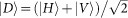is then prepared for both photons using a half wave-plate with optic axis set to π/8 radians. The polarisation in the left arm is matched and purified to that of the right using a rotated polarisation beam splitter in transmission. The two photon state launched into the waveguide array is then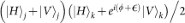. The collecting PMF and the birefringence of the waveguide array preserve horizontal and vertical polarisation components for both photons and prohibit crosstalk between horizontal and vertical light; PMF-coupled polarisation beam splitters at the output of the waveguide array allow two-photon correlated detections across the two orthogonal polarisations to project the initial input state to be the entangled state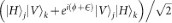; the extra phaseis compensated using a Z(θ) rotation on the right arm of the photon source comprised of two quarter waveplates (optic axis at π/4 radians) with a half waveplate between (optic axis at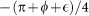radians, see Fig. 1 of the main text).

The waveguide array was fabricated from silicon oxynitride (SiON) thin films were grown by plasma enhanced chemical vapour deposition (PECVD) onto thermally oxidised <100> oriented 100-mm silicon wafers applying an Oxford 80 Plasmalab Systems. A deposition process based on 2% SiH4/N2 and N2O was performed at 60 W power, 187.5 kHz frequency, 650 mTorr pressure and 300°C substrate temperature. The SiON films were annealed for 3 hours at 1150°C in a nitrogen atmosphere. By optical inspection at 632 nm wavelength a film thickness of d = 603 (±3) nm and a refractive index (for TE) of nTE = 1.5232 (±1 × 10−3) were measured. The channel waveguides were obtained from standard lithography followed by reactive ion etching in CHF3/O2 chemistry at 350 W plasma power. The etch depth (d = 613 ± 5 nm) was confirmed by profilometry. A 5-μm thick upper cladding layer was deposited in two steps applying a Low Pressure CVD TEOS-process and standard PECVD SiO2 deposition. The upper cladding was annealed at 1150°C for 3 hours. For a core width of 1.8-μm the total birefringence of the waveguide, including material and form birefringence34, is of the order Δn = nTMnTE = 2 * 10−4. This value provides polarization preserving propagation for the TE and TM photons throughout the device. Moreover, the birefringence is small enough to ensure a nearly identical mode overlap and coupling ratio, between different waveguides in the coupled array for TM and TE modes. This implies that the processes Aa = ATE and Ab = A™ are matched, as required and verified in the reported experiment. The coupled waveguide arrays were accessed with lithographically patterned bends (minimum bend radius of 600 μm) that gradually mapped the 250 μm pitched input (for 250 μm pitched PMF array access) to the 2.8 μm pitch in the evanescent coupling region. The coupling region is 350 μm long, before bending gradually to an output pitch of 125 μm which was accessed by 250 μm pitched arrays of PMF; the output measurements were alternated between access to all odd labeled waveguides and all even labeled waveguides. The optical chips were diced from the wafer.

## Change history

• ### 18 April 2013

A correction has been published and is appended to both the HTML and PDF versions of this paper. The error has been fixed in the paper.

## References

• Hong, C. K., Ou, Z. Y. & Mandel, L. Measurement of subpicosecond time intervals between two photons by interference. Phys. Rev. Lett. 59, 2044–2046 (1987).

• Liu, R. C., Odom, B., Yamamoto, Y. & Tarucha, S. Quantum interference in electron collision. Nature 391, 263–265 (1998).

• Wilczek, F. Magnetic flux, angular momentum and statistics. Phys. Rev. Lett. 48, 1144–1146 (1982).

• Wilczek, F. Fractional statistics and anyon superconductivity. World Scientific, (1990).

• Laughlin, R. B. Anomalous quantum hall effect: An incompressible quantum fluid with fractionally charged excitation. Phys. Rev. Lett. 50, 1395–1398 (1983).

• Zeilinger, A. Quantum entanglement: a fundamental concept finding its applications. Physica Scripta T 76, 203–209 (1998).

• Michler, M., Mattle, K., Weinfurter, H. & Zeilinger, A. Interferometric bell-state analysis. Phys. Rev. A 53, R1209–R1212 (1996).

• Wang, Q., Zhang, Y.-S., Huang, Y.-F. & Guo, G.-C. Simulating the fourth-order interference phenomenon of anyons with photon pairs. Eur. Phys. J. D 42, 179–182 (2007).

• Rarity, J. G. et al. Two-photon interference in a mach-zehnder interferometer. Phys. Rev. Lett. 65, 1348–1351 (1990).

• Perets, H. B. et al. Realization of quantum walks with negligible decoherence in waveguide lattices. Phys. Rev. Lett. 100, 170506 (2008).

• Peruzzo, A. et al. Quantum walks of correlated photons. Science 329, 1500–1503 (2010).

• Hines, A. P. & Stamp, P. C. E. Quantum walks, quantum gates and quantum computers. Phys. Rev. A 75, 062321 (2007).

• Lim, Y. L. & Beige, A. Generalized hong-ou-mandel experiments with bosons and fermions. New J. Phys. 7, 155 (2005).

• Bromberg, A., Lahini, Y., Morandotti, R. & Silberberg, Y. Quantum and classical correlations in waveguide lattices. Phys. Rev. Lett. 102, 253904 (2009).

• Kassal, I., Whitfield, J. D., Perdomo-Ortiz, A., Yung, M.-H. & Aspuru-Guzik, A. Simulating chemistry using quantum computers. Annu. Rev. Phys. Chem. 62, 185–207 (2011).

• Abrams, D. S. & Lloyd, S. Simulation of many-body fermi systems on a universal quantum computer. Phys. Rev. Lett. 79, 2586–2589 (1997).

• Ward, N. J., Kassal, I. & Aspuru-Guzik, A. Preparation of many-body states for quantum simulation. J. Chem. Phys. 130, 194105 (2009).

• Knill, E., Laflamme, R. & Milburn, G. J. A scheme for efficient quantum computation with linear optics. Nature 409, 46–52 (2001).

• Scheel, S. Permanents in linear optical networks. arXiv:quant-ph/0406127v1 (2004).

• Aaronson, S. & Arkhipov, A. The computational complexity of linear optics. Proceedings of the 43rd annual ACM symposium on Theory of computing, 333–342 (2011).

• Aaronson, S. & Arkhipov, A. The computational complexity of linear optics. arXiv:1011.3245 [quant-ph] (2010).

• Politi, A., Cryan, M. J., Rarity, J. G., Yu, S. & O'Brien, J. L. Silica-on-silicon waveguide quantum circuits. Science 320, 646–649 (2008).

• Matthews, J. C. F., Politi, A., Bonneau, D. & O'Brien, J. L. Heralding two-photon and four-photon path entanglement on a chip. Phys. Rev. Lett. 107, 163602 (2011).

• Peruzzo, A., Laing, A., Politi, A., Rudolph, R. & O'Brien, J. L. Multimode quantum interference of photons in multiport integrated devices. Nature Communications 2, 224 (2011).

• Rom, T. et al. Free fermion antibunching in a degerenate atomic fermi gas released from an optical lattice. Nature 444, 733–736 (2006).

• Harrison, J. M., Keating, J. P. & Robbins, J. M. Quantum statistics on graphs. Proc. R. Soc. A 467, 212–233 (2011).

• Keil, E. et al. Photon correlations in two-dimensional waveguide arrays and their classical estimate. Phys. Rev. A 81, 023834 (2010).

• Keilmann, T., Lanzmich, S., McCulloch, I. & Roncaglia, M. Statistically induced phase transitions and anyons in 1d optical lattices. Nature Communications 2, 361 (2011).

• Lu, M. et al. Demonstrating anyonic fractional statistics with a six-qubit quantum simulator. Phys. Rev. Lett. 102, 030502 (2009).

• Pachos, J. K. et al. H. Weinfurter. Revealing anyonic features in a toric code quantum simulation. New J. Phys. 11, 083010 (2009).

• Reck, M., Zeilinger, A., Bernstein, H. J. & Bertani, P. Experimental realization of an discrete unitary operator. Phys. Rev. Lett. 73, 58–61 (1994).

• Matthews, J. C. F., Politi, A., Stefanov, A. & O'Brien, J. L. Manipulation of multiphoton entanglement in waveguide quantum circuits. Nat. Photonics 3, 346–350 (2009).

• Okamoto, R., O'Brien, J. L., Hofmann, H. F. & Takeuchi, S. Realization of a knill-laflamme-milburn controlled-not photonic quantum circuit combining effective optical nonlinearities. Proceedings of the National Academy of Science 108, 10067–10071 (2011).

• Wörhoff, K., Roeloffzen, C. G. H., de Ridder, R. M., Driessen, A. & Lambeck, P. V. Design and Application of Compact and Highly Tolerant Polarization-Independent Waveguides. J. Lightw. Technol. 75, 1276–1283 (2007).

## Acknowledgements

This work was supported by EPSRC, ERC, US AFOSR, NSQI and European projects PHORBITECH, QUANTIP. J.C.F.M. is supported by a Leverhulme Trust Early Career Fellowship. A.Pe. holds a Royal Academy of Engineering Research Fellowship. J.L.O.B. acknowledges a Royal Society Wolfson Merit Award and a Royal Academy of Engineering Chair in Emerging Technologies.

## Author information

Authors

### Contributions

J.C.F.M., K.P., J.D.A.M. and A.P. contributed equally to writing this manuscript and conducting the experiment. All authors reviewed the manuscript.

## Ethics declarations

### Competing interests

The authors declare no competing financial interests.

## Electronic supplementary material

### Supplementary Information

Supplementary information for: Observing fermionic statistics with photons in arbitrary processes

## Rights and permissions

Reprints and Permissions

Matthews, J., Poulios, K., Meinecke, J. et al. Observing fermionic statistics with photons in arbitrary processes. Sci Rep 3, 1539 (2013). https://doi.org/10.1038/srep01539

• Accepted:

• Published:

• DOI: https://doi.org/10.1038/srep01539

• ### Birefringence aids photonic lattice simulations

• Hao Tang
• Xian-Min Jin

Nature Photonics (2022)

• ### A non-unitary metasurface enables continuous control of quantum photon–photon interactions from bosonic to fermionic

• Quanwei Li
• Wei Bao
• Xiang Zhang

Nature Photonics (2021)

• ### Asymptotic Gaussian law for noninteracting indistinguishable particles in random networks

• Valery S. Shchesnovich

Scientific Reports (2017)

• ### Experimental perfect state transfer of an entangled photonic qubit

• Robert J. Chapman
• Matteo Santandrea
• Alberto Peruzzo

Nature Communications (2016)

• ### High-dimensional entanglement certification

• Zixin Huang
• Lorenzo Maccone
• Alberto Peruzzo

Scientific Reports (2016)